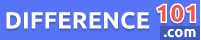# Bar Graph vs. Histogram: What Is the Difference Between Bar Graph and Histogram?

Bar graphs and histograms are two ways of representing data, and they are often used as a tool for statistical analysis and comparison. Read more to find out their differences.After collecting the data, it is very important to display it in a specific way to highlight its features. For that purpose, diagrammatic representation is the best way of representing the data. A histogram and bar graph are two ways in which the data can be displayed in the form of a diagram. Both of these terms use bars to display data. The main difference between bar graph and histogram is in the way they represent the data.

Let’s take a closer look at the Bar Graph vs. Histogram

## What is a Bar Graph?

The bar graph is a chart that is used to represent the comparison of categorized data. It has parallel rectangular bars which have the same width but different lengths. These bars display the grouped data. Each rectangular block represents a specific category, and they are arranged in such a way in a bar that they do not touch each other. Bar diagrams can be both vertical and horizontal. It contains two axes: one axis displays the categories while the other is to display the discrete value of data.

👉  Seminar vs. Lecture: What Is the Difference Between Seminar and Lecture?

## What is Histogram?

The histogram can be defined as a bar chart, which shows the frequency distribution of continuous data with the help of bars to represent the statistical information. It shows the observation present in-between the range of values, called class or bin. It is the first step of constructing the histogram to take the observation and split these into intervals called bins. It has two axis, x and y. the x-axis shows the independent variables while the y-axis shows dependent variables.

## Bar Graphs vs. Histograms Similarities

• Histograms and bar graphs both depend upon bars to represent and display the data.
• Whether it is a histogram or bar chart, both have a two-axis, x-axis, and y-axis.

## Pros and Cons of Bar Graph

### Pros of Bar Graph

• A bar graph can summarize a large amount of data visualization to make it easier to understand.
• Can easily compare more than one data set and make quick and accurate estimates.
• It is the best method for representing the data and is used by many industries.
👉  Mcafee Livesafe vs. Total Protection: What is the difference between McAfee livesafe and Total protection?

### Cons of Bar Graph

• It often needs additional explanation and cannot expose the causes, patterns, and impacts.
• Bar graphs use only discrete data.
• Don’t show a relationship between activities, making it difficult to manage projects.

## Pros and Cons of Histogram

### Pros of Histogram

• Histograms are used very large in marketing campaigns and project management for visualization purposes.
• Another advantage of the histogram is that it can represent a large number of bars, which represent different class intervals.
• A single glance at a histogram can give an idea about the shape and structure of the data.

### Cons of Histogram

• A histogram cannot display discrete data. It can only show the continuous frequency distribution.
• Histograms cannot provide information like upper quartile, lower quartile, and median of data.

## Bar Graph vs. Histogram FAQs

### Is A Histogram a Bar Graph?

Yes, a histogram is basically a type of bar graph used to display quantitative data. The histogram shows the frequency of non-discrete data.

### How is a Histogram Different From A Bar Graph?

Histograms and bar graphs are different from each other as histograms display quantitative data while bar graphs display categorical data.

### When to Use Histogram vs. Bar Graph?

Histograms are used when you need to show the distribution of variables, and the bar graph is used when you need to compare variables.

### Can Histograms Have Gaps?

A histogram resembles a bar graph, but it does not have any gaps between the bars.

## Conclusion

If we look at histogram Versus bar graph, Both are used to display data with the help of bars, and both have x and y-axis. The height of the bars depends upon the frequency of the data in the element. But the main difference between bar graphs and histograms is  in presentation as bar graphs represent categorical data while histograms represent quantitative data. These are also different in the arrangement of bars. The bars of the bar graph have space between them and cannot touch each other. But the histogram bars don’t have any space and touch each other.

👉  Graphic Card vs. Video Card: What Is the Difference Between Graphic Card and Video Card?
We will be happy to hear your thoughtsLime vs. Lemon: What’s the difference?